• Call Now

1800-102-2727•

# Area of Trapezium Definition, Types, Properties, Derivation and FAQs

A Trapezium is a quadrilateral in a two-dimensional space with one pair of parallel sides. It is a convex figure which means that it is a closed figure with all its interior angles less than 180 and vertices pointing outwards. It has four sides and four vertices. Since it has only one pair of parallel sides, the other two are non-parallel sides and are called legs and the parallel sides are called bases. The illustration of the trapezium is given below. In this article, we will learn how to find the area of a trapezium with the help of some practice problems.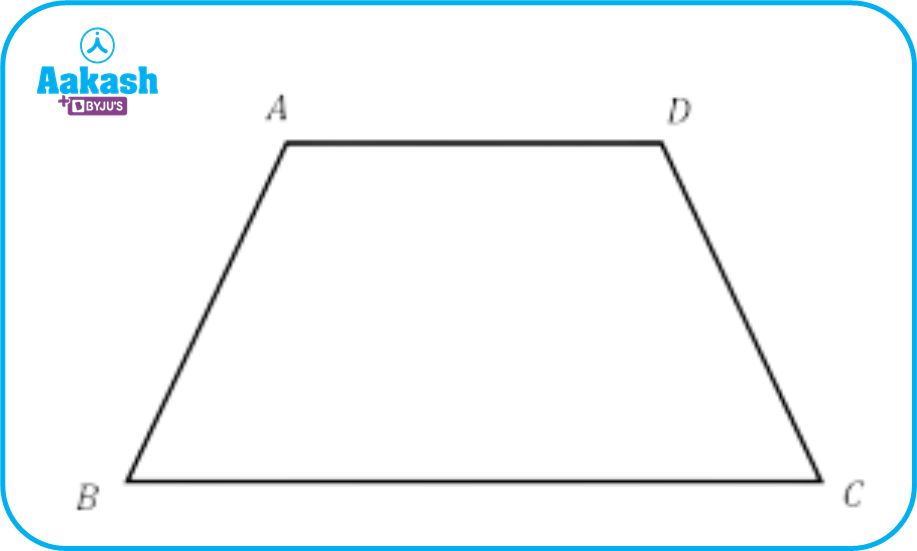• Definition of Area of Trapezium
• Types
• Scalene Trapezium
• Isosceles Trapezium
• Right Trapezium
• Properties of Trapezium
• Derivation of Area of Trapezium
• Practice Problems
• FAQs

## Definition of Area of Trapezium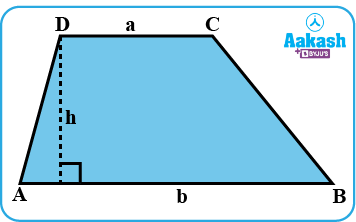The area of the trapezium or trapezoid is the space covered by the trapezium on a two-dimensional plane. A trapezium is a quadrilateral with four sides and four vertices. The area of trapezium is the half of the sum of the parallel sides times the height of the trapezium.Area of Trapezium =Area of Trapezium =

Here, a and b are bases.

And h is the height or perpendicular distance between a and b.

## Types

There are three types of trapeziums:

1. Scalene Trapezium
2. Isosceles Trapezium
3. Right Trapezium

Let’s discuss these in brief one by one.

### Scalene Trapezium

The word ‘scalene’ means ‘unequal’. The scalene trapezium is the trapezium that has all its sides and angles of different measures.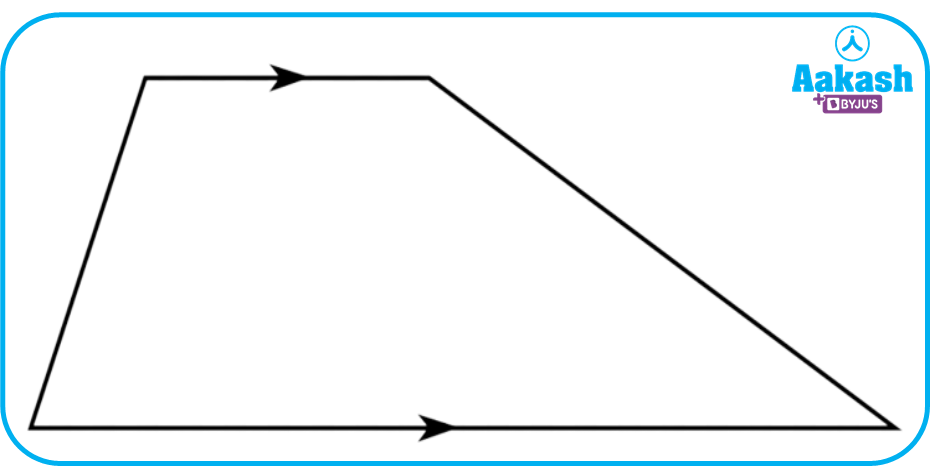Scalene Trapezium

### Isosceles Trapezium

The word Isosceles means ‘equal leg’. The trapezium is called isosceles trapezium when both of its legs or non-parallel sides are equal.Isosceles Trapezium

### Right Trapezium

A trapezium is said to be a right trapezium when at least one angle of a trapezium is a right angle.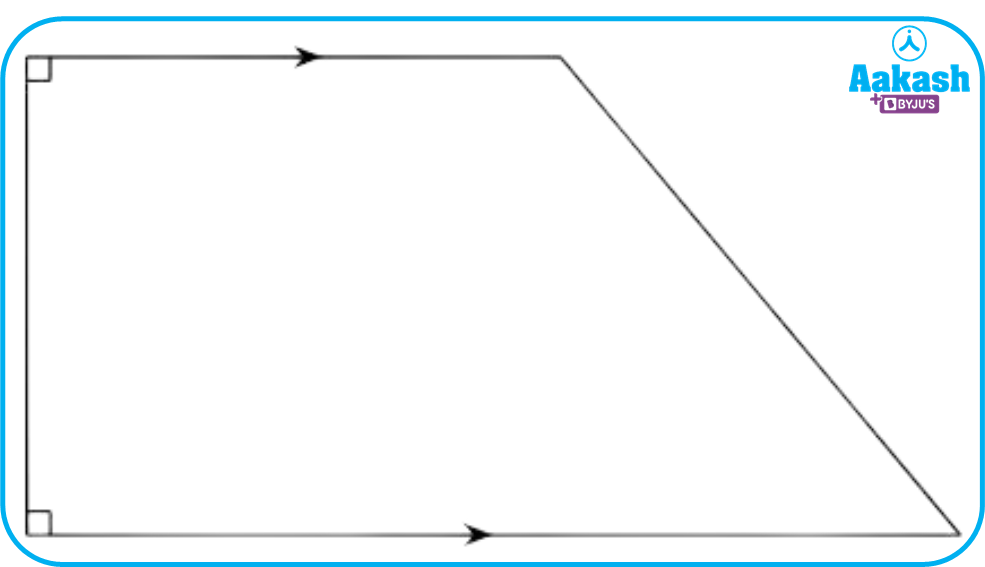Right Trapezium

## Derivation of Area of Trapezium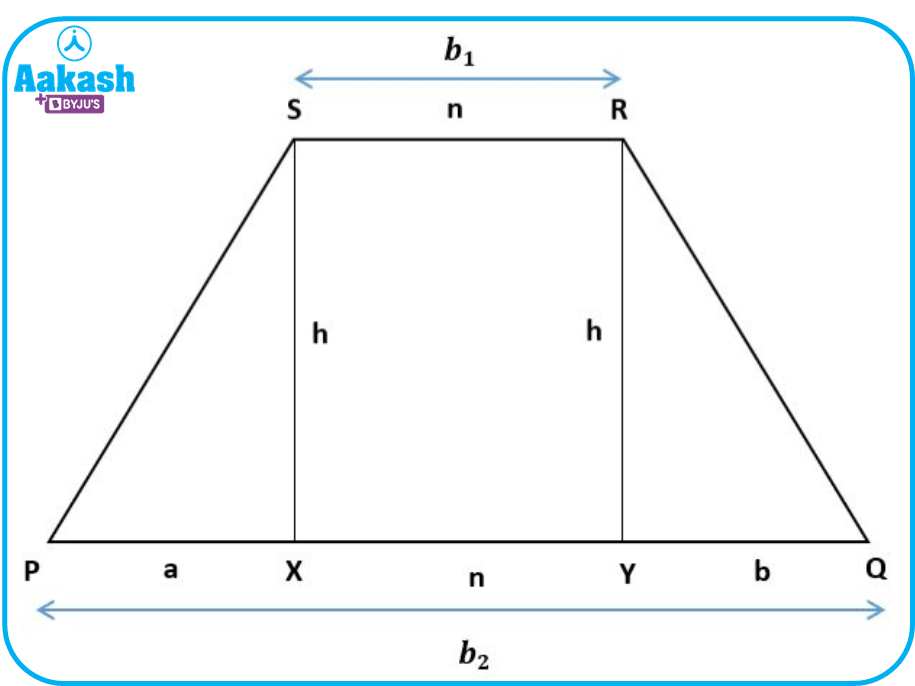Now, let us learn how to derive the formula for the area of the trapezium.

From the above diagram, we can see that trapezium PQRS comprises of Δ PXS, Δ QYR and rectangle XYRS.

In Δ PXS, the base is a and height is h.

In Δ QYR, the base is b and height is h.

In the rectangle XYRS, length is n and width is h.

Also,

${b}_{1}=n$

${b}_{2}=a+n+b$

Now, the area of trapezium is equal to the sum of the area of two triangles and the area of the rectangle.

Area of PQRS=Area of Δ PXS+Area of Δ QYR+Area of XYRSSo, the area of a trapezium with bases b1 and b2 and height h is given by

## Practice Problems

1. Find the area of trapezium when the sum of the parallel sides is 20 cm and the height is 5 cm.

Solution: Given: b1+b2=20 cm and h=5 cm

Area = ?

Area of a Trapezium =

2. Find the distance between the parallel sides of the trapezium when the length of the parallel sides are 15 cm and 18 cm and the area is 99 cm2.

Solution: Given: Area of a Trapezium = 99 cm2

b1=15 cm and b2=18 cm

h= ?

Now, Area of a Trapezium

3. Find the area of the trapezium given below.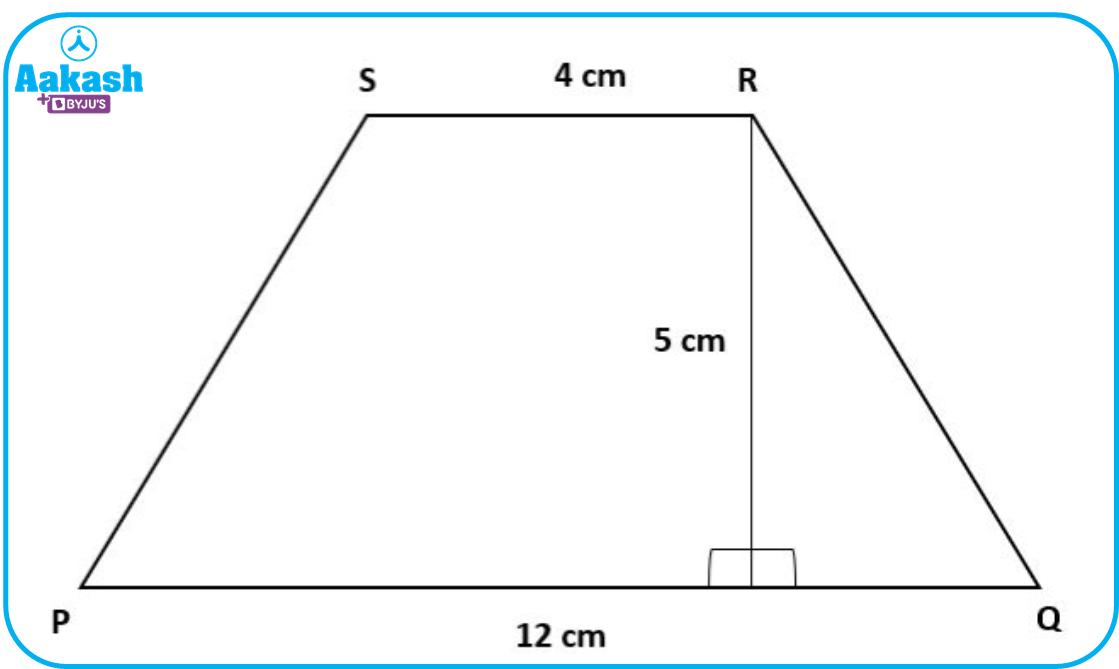Solution: Given: b1=4 cm , b2=12 cm and h=5 cm

Area of given trapezium =

4. Calculate the area of a trapezium whose parallel sides are 45.7 cm and 68.3 cm and the height is 5 cm.

Solution:

Now, Area of a Trapezium

#### 5. Find the area of the trapezium when the length of parallel sides is given as 10 cm and 25 cm and the length of non-parallel sides is given as 13 cm and 14 cm.

Solution: Let ABCD be a trapezium in which

AB=10 cm, BC=14 cm, CD=25 cm and DA=13 cm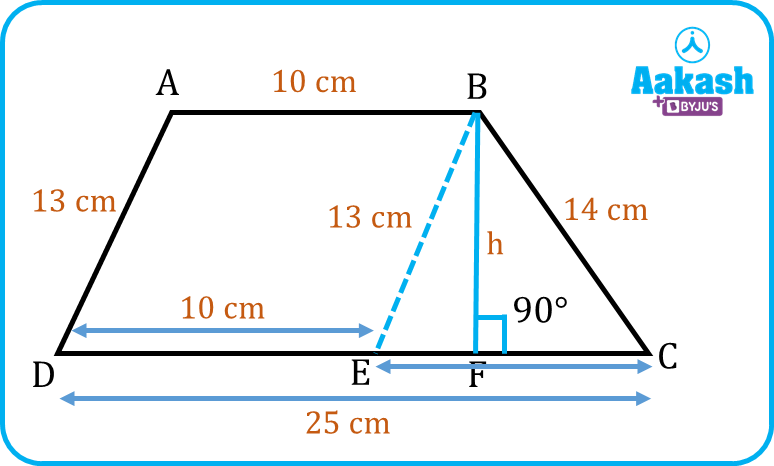Construct a line from a point B parallel to side AD, which cuts CD at E.

As we know that, Area of trapezium =h2(a+b) square units

But, for that, we have to find the height or distance between parallel sides.

Now, in CBE, we apply Heron’s formula

$A=\sqrt{s\left(s-a\right)\left(s-b\right)\left(s-c\right)}=\sqrt{21\left(21-13\right)\left(21-14\right)\left(21-15\right)}$

Also,

Now, the area of the trapezium is,

Hence, the area of the trapezium

1. What is an irregular trapezium?
A.
A trapezium having its non - parallel sides of unequal length is said to be an irregular trapezium.

2. What is the height of a trapezium?
A.
The perpendicular distance between the parallel sides of a trapezium is the height of a trapezium.

3. What is right trapezium?
A.
A trapezium having two right angles is called a right trapezium.

4. Does a trapezium have equal diagonals?
A.
Yes, the trapezium can have equal diagonals when the given trapezium is an isosceles trapezium.Talk to our expert
Resend OTP Timer =
By submitting up, I agree to receive all the Whatsapp communication on my registered number and Aakash terms and conditions and privacy policy